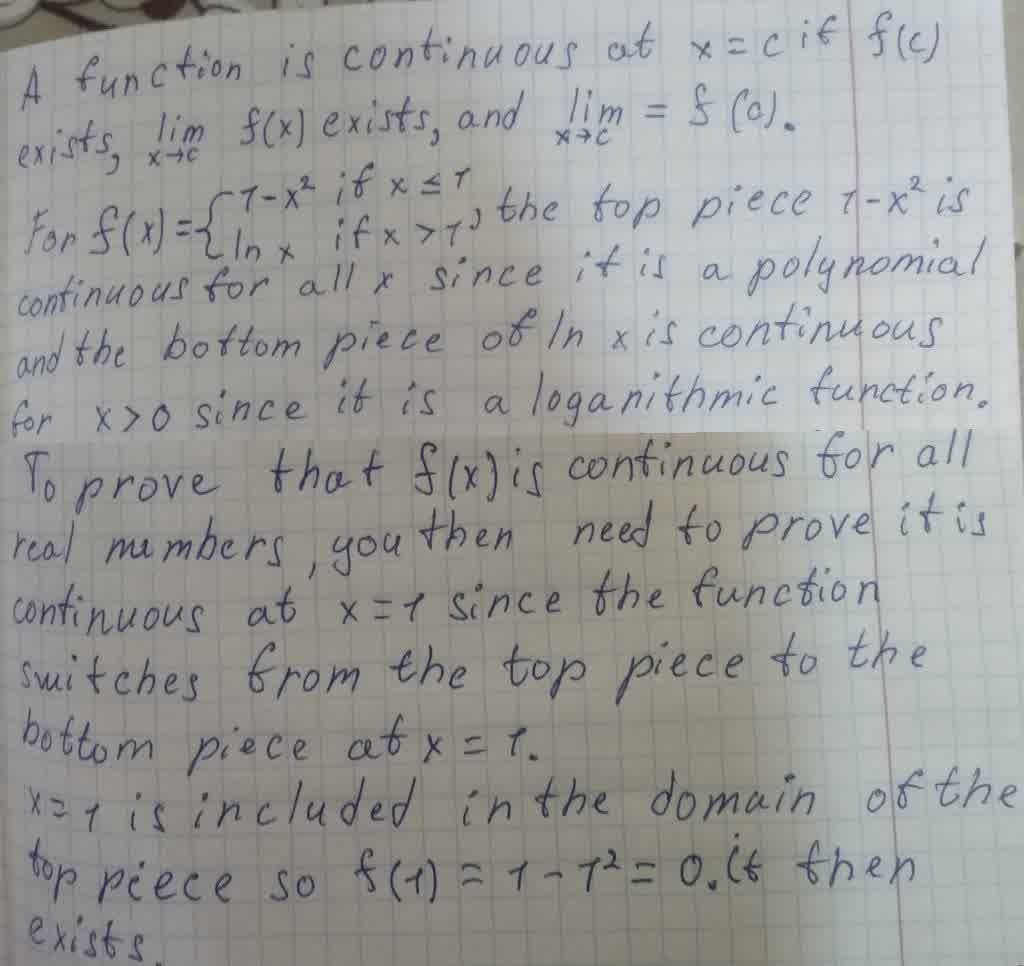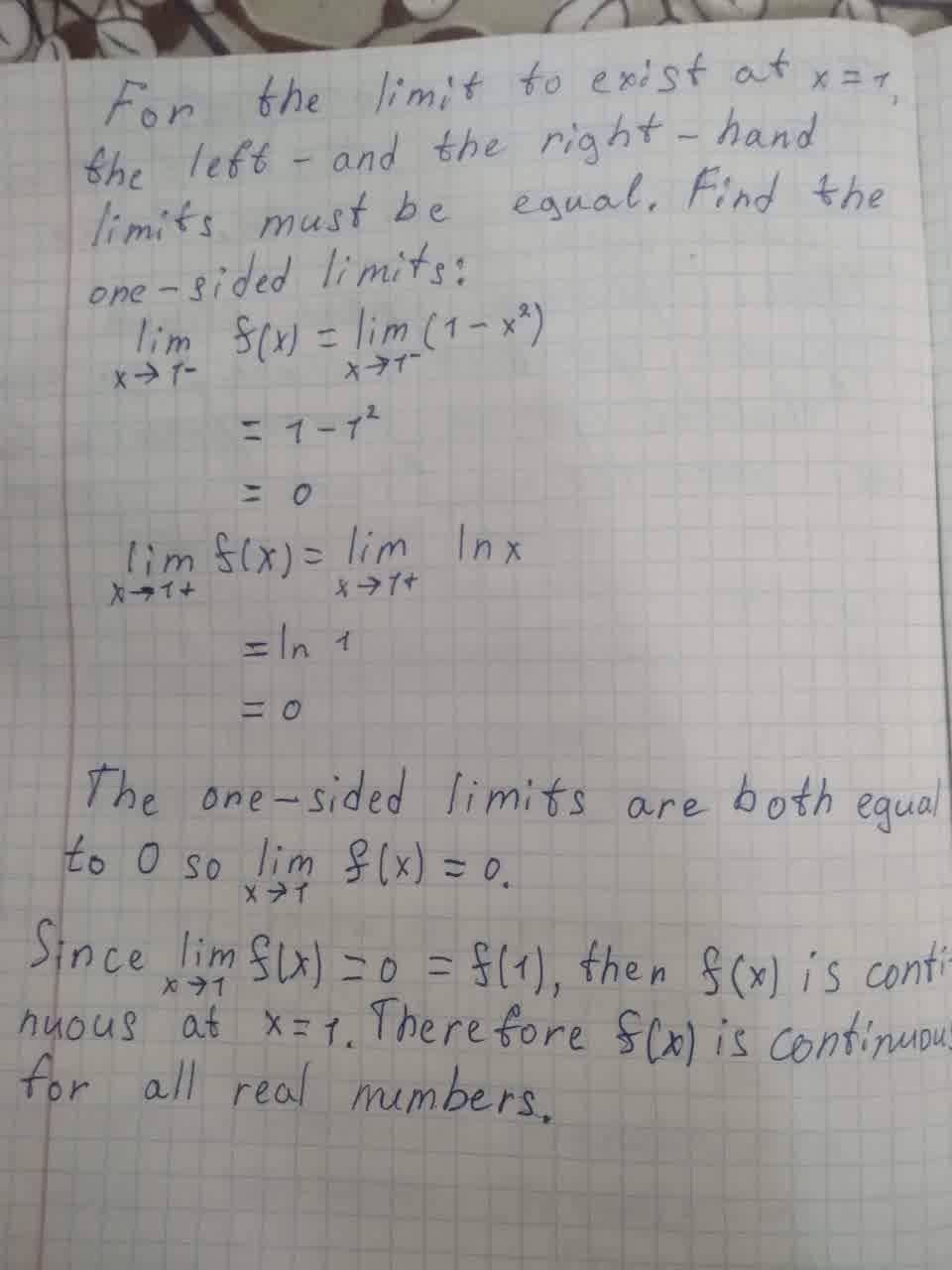Question# Show that f is continuous on (−\infty, \infty) f(x) = 1 − x^2 if x \leq 1 ln(x) if x > 1

Polynomial arithmetic
ANSWEREDShow that f is continuous on $$\displaystyle{\left(−\infty,\infty\right)}$$.
f(x) =
$$\displaystyle{1}−{x}^{{2}}{\quad\text{if}\quad}{x}\leq{1}$$
ln(x) if x > 1